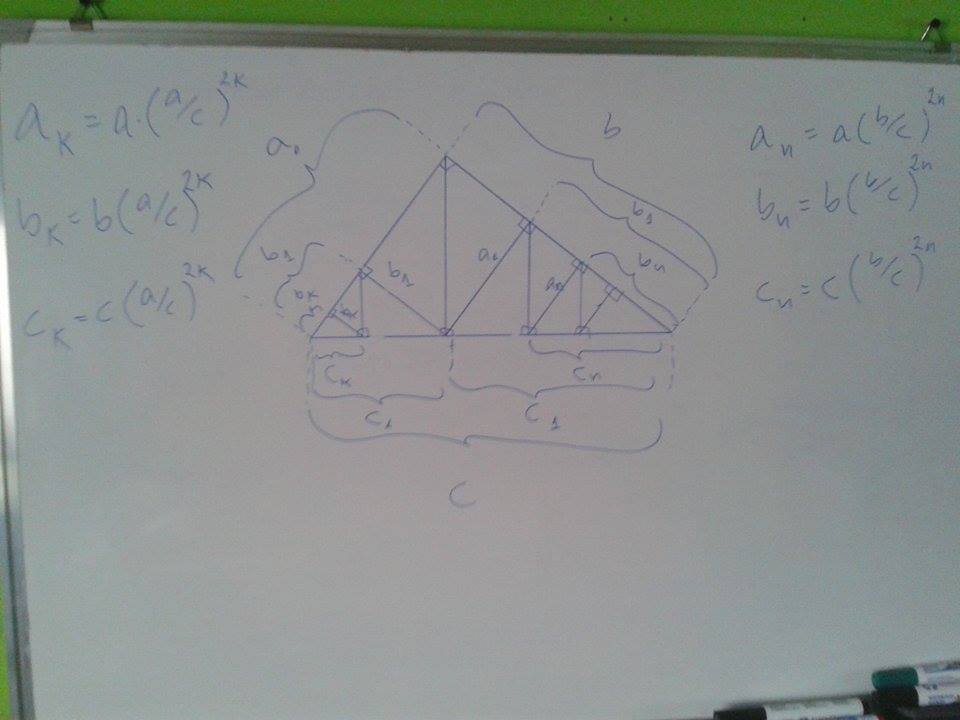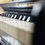# A Big Doubt (Own Theorem)

A few years ago I discovered this property and it supposed to be my own Geometry Theorem. I want to know if is it real. Have you seen a Theorem like this?As a corollary we can say:

If we let $a$ and $b$ be the foots of a right triangle with hypotenuse $c$, we have

$\dfrac {c^{2}}{a^{2}} = \sum_{n=0}^\infty (\dfrac{b}{c})^{2n}$ And $\dfrac{c^{2}}{b^{2}}=\sum_{n=0}^\infty (\dfrac{a}{c})^{2n}$Note by Hjalmar Orellana Soto
6 years ago

This discussion board is a place to discuss our Daily Challenges and the math and science related to those challenges. Explanations are more than just a solution — they should explain the steps and thinking strategies that you used to obtain the solution. Comments should further the discussion of math and science.

When posting on Brilliant:

• Use the emojis to react to an explanation, whether you're congratulating a job well done , or just really confused .
• Ask specific questions about the challenge or the steps in somebody's explanation. Well-posed questions can add a lot to the discussion, but posting "I don't understand!" doesn't help anyone.
• Try to contribute something new to the discussion, whether it is an extension, generalization or other idea related to the challenge.

MarkdownAppears as
*italics* or _italics_ italics
**bold** or __bold__ bold
- bulleted- list
• bulleted
• list
1. numbered2. list
1. numbered
2. list
Note: you must add a full line of space before and after lists for them to show up correctly
paragraph 1paragraph 2

paragraph 1

paragraph 2

[example link](https://brilliant.org)example link
> This is a quote
This is a quote
    # I indented these lines
# 4 spaces, and now they show
# up as a code block.

print "hello world"
# I indented these lines
# 4 spaces, and now they show
# up as a code block.

print "hello world"
MathAppears as
Remember to wrap math in $$ ... $$ or $ ... $ to ensure proper formatting.
2 \times 3 $2 \times 3$
2^{34} $2^{34}$
a_{i-1} $a_{i-1}$
\frac{2}{3} $\frac{2}{3}$
\sqrt{2} $\sqrt{2}$
\sum_{i=1}^3 $\sum_{i=1}^3$
\sin \theta $\sin \theta$
\boxed{123} $\boxed{123}$

Sort by:

You would need to explain the set up with some words, in order to explicitly convey what you intend.

Staff - 6 years ago

For the triangle in the above photo we know by euclidean theorem that $a^{2}=pc$, and $h=\frac{ab}{c}$. So it is true that - $a_{1} = \frac{c_{1}h}{b}$ - $a_{1}=\frac{\frac{b^{2}}{c}\frac{ab}{c}}{b}=a(\frac{b}{c})^{2}$ It's easy to see that $c_{1}=c(\frac{b}{c})^{2}$ by euclidean theorem. And the same with $b_{1}$ to get $b_{1}=b(\frac{b}{c})^{2}$. If you take it with the next triangles at that side you'll find that $a_{n}=a(\frac{b}{c})^{2n}$, $b_{n}=b(\frac{b}{c})^{2n}$ and $c_{n}=c(\frac{b}{c})^{2n}$ And with the triangles at the other side you'll find that $a_{k}=a(\frac{a}{c})^{2k}$, $b_{k}=b(\frac{a}{c})^{2k}$ and $c_{k}=c(\frac{a}{c})^{2k}$

My calculations in Maple show me that it's true for triangles :

(a=4, b = 8.01, c = 8.95)

(a=3, b = 4, c = 5)

(a=4.9875077, b=12.429690, c=13.392999)

- 6 years ago

The corollary or the theorem?

I think it's theorem, I have not seen it before.

- 6 years ago

I've seen to problem of this sort. The closed form of $a_{k}$ wasn't given, but we had to sum a very similar series.

- 5 years, 4 months ago